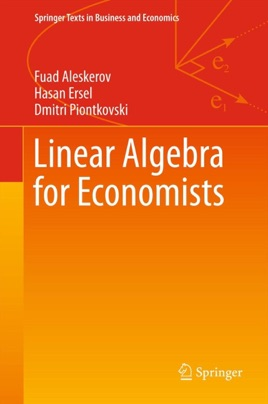• \$64.99

## Publisher Description

This textbook introduces students of economics to the fundamental notions and instruments in linear algebra. Linearity is used as a first approximation to many problems that are studied in different branches of science, including economics and other social sciences. Linear algebra is also the most suitable to teach students what proofs are and how to prove a statement. The proofs that are given in the text are relatively easy to understand and also endow the student with different ways of thinking in making proofs. Theorems for which no proofs are given in the book are illustrated via figures and examples. All notions are illustrated appealing to geometric intuition. The book provides a variety of economic examples using linear algebraic tools. It mainly addresses students in economics who need to build up skills in understanding mathematical reasoning. Students in mathematics and informatics may also be interested in learning about the use of mathematics in economics.